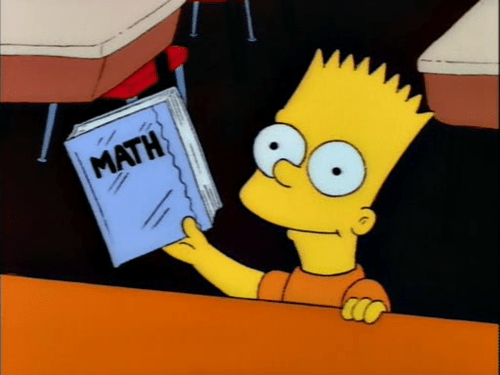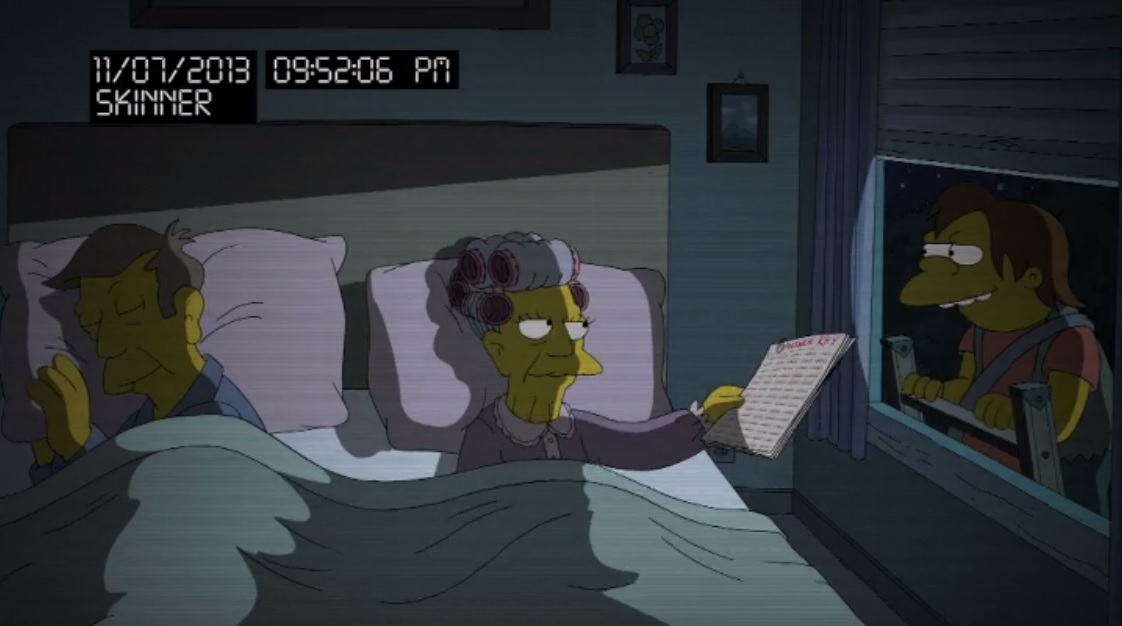# Super Safi’s Monday Morning Math Mayhem 26 – Reducing Fractions

Morning Mathematical Monsters & Maniacs!

(Today’s post is sponsored by the letter “M”)

Hi, I’m Super Safi and you may remember me from such stats and strategy posts as Kwik-E-Mart Farming and the advanced losing-to-win Superheroes battle strategy.

Over the past 600+ episodes, The Simpsons has taken us on an amazing mathematical journey involving fractions, probability, Fermat’s last theorem, and hundreds of other aspects from the wonderful world off mathematics.

And what better way to start your week, then by discussing math Monday morning?This week we look at Bart’s homework on reducing fractions to irreducible fractions. I know this will split you readers into either Barts or Lisas from Postcards From the Wedge (Season 21, E14), as they so eloquently discussed fractions:

Bart:  “I would end all life on this planet just to get out of doing fractions.
Lisa:  “Fractions aren’t that hard. You just have to have a common denominator.  For example, one-half plus one-third equals three-…
Bart:  “End. all. life. on. this. planet!” [makes explosion sound]
Lisa:  “You’ll need to know fractions to make that explosion!

So let’s find out if you’re a Bart or a Lisa.

In YOLO (Season 25, Episode 04), Kent Brockman and his crew visit Springfield Elementary to reveal the rampant cheating students are partaking in, with a little help from Principal Skinner’s own mother:Lisa has a solution, she creates the school honour code, where every student promises not to cheat and report every cheater they see. The parents quickly agree to Lisa’s idea:However, Lisa soon realizes Bart is cheating, when she discovers his math homework on reducing fractions:Irreducible Fractions

An irreducible fraction (or reduced fraction) is a fraction in which the numerator and denominator are integers that have no other common divisors than 1.

So let’s look at Bart’s math test.

For those who may have forgotten our discussion on Euclidean algorithms as an efficient method for computing the greatest common divisor (GCD) of two numbers, please click here on Math Mayhem 19 first.

1. 28/42

So to calculate the GCD of 28 and 42, we start by calculating how many times 28 can go into 42 and how much remainder is leftover.

42 = 1 x 28 + 14

Next we calculate how many times that remainder 14 can go into 28 and how much remainder is leftover.

28 = 2 x 14 + 0

As the remainder is 0, we can conclude 14 is the greatest common divisor. So to reduce the fraction 28/42, we divide both the numerator and denominator by the greatest common divisor.

28/42 can be reduced by a GCD of 14 to 28 ÷ 14 over 42 ÷ 14 = 2/3

2. 24/40

So to calculate the GCD of 24 and 40, we start by calculating how many times 24 can go into 40 and how much remainder is leftover.

40 = 1 x 24 + 16

Next we calculate how many times that remainder 16 can go into 24 and how much remainder is leftover.

24 = 1 x 16 + 8

So next we calculate how many times that remainder 8 can go into 16 and how much remainder is leftover.

16 = 2 x 8 + 0

As the remainder is 0, we can conclude 8 is the greatest common divisor. So to reduce the fraction 24/40, we divide both the numerator and denominator by the greatest common divisor.

24/40 can be reduced by a GCD of 8 to 24 ÷ 8 over 40 ÷ 8 = 3/5

3. 22/66

So to calculate the GCD of 22 and 66, we start by calculating how many times 22 can go into 66 and how much remainder is leftover.

66 = 3 x 22 + 0

As the remainder is 0, we can conclude 22 is the greatest common divisor. So to reduce the fraction 22/66, we divide both the numerator and denominator by the greatest common divisor.

22/66 can be reduced by a GCD of 22 to 22 ÷ 22 over 66 ÷ 22 = 1/3

4. 15/20

So to calculate the GCD of 15 and 20, we start by calculating how many times 15 can go into 20 and how much remainder is leftover.

20 = 1 x 15 + 5

Next we calculate how many times that remainder 5 can go into 15 and how much remainder is leftover.

15 = 3 x 5 + 0

As the remainder is 0, we can conclude 5 is the greatest common divisor. So to reduce the fraction 15/20, we divide both the numerator and denominator by the greatest common divisor.

15/20 can be reduced by a GCD of 5 to 15 ÷ 5 over 20 ÷ 5 = 3/4

5. 12/36

So to calculate the GCD of 12 and 36, we start by calculating how many times 12 can go into 36 and how much remainder is leftover.

36 = 3 x 12 + 0

As the remainder is 0, we can conclude 12 is the greatest common divisor. So to reduce the fraction 12/36, we divide both the numerator and denominator by the greatest common divisor.

12/36 can be reduced by a GCD of 12 to 12 ÷ 12 over 36 ÷ 12 = 1/3

Now that we’ve completed a look at Bart’s fraction homework, why not show your love for math and fractions with your own Math Mayhem shirt or hoodie.

If you love math or enjoy reading these posts, don’t forget to stop by the Addicts Shop and check out all the paraphernalia, including the Math Mayhem shirts and hoodies.Were you familiar with reducing fractions? Did you attempt to do the math yourself? Are you a Bart or a Lisa when it comes to fraction appreciation? Do you remember these two episodes from the show? Sound off in the comments below. You know we love hearing from you.

### 18 responses to “Super Safi’s Monday Morning Math Mayhem 26 – Reducing Fractions”

1.ibuylow2014

In my 40+ years as an engineer, I can say I never needed to know the GCD of a fraction.

•Safi

Ah, so you’re the engineer responsible for that bridge that collapsed. Should have used the GCD. 🙂

2.Charles

Seems like the long way around it. Easiest way is to use common factors.
For example 12/36. 12 = 6*2. 36 = 6*6. Thus 6*2/6*6. Since n/n =1, reduces to 2/6 = 2/2*3 = 1/3.

•Graham-S

Why muck about, I see straight off your example is 1×12 / 3×12 but how would your method cope with more complex numbers such as 1551 / 6721 ??

•Charles

1551 / 6721?
They are not even, but the top is divisible by 3, so we get 3*517. Using 517, we find 6721/517 = 13. So 3*517/13*517 = 3/13.

•Graham-S

Yup.

•Graham-S

Safi’s method for the calculator generation :-
6721 / 1551 = 4.3..
6721 – ( 4 x 1551 ) = 517
1551 / 517 = 3
6721 / 517 = 13

•Charles

Try 35/81.

•Graham-S

That is as simplified as it gets, but I went and did the math to show it anyway

81 / 35 = 2.31..
81 – ( 2 x 35 ) = 11
35 / 11 = 3.18..
35 – ( 3 x 11 ) = 2
11 / 2 = 5.5
11 – ( 5 x 2 ) = 1

2 / 1 = 2

35 / 1 = 35
81 / 1 = 81

•Charles

versus
35/81
5 is a factor of 35, leaving 35/7 = 7.
81/7 = 11.5…(thus not divisible by 7)
thus 35/81, a lot quicker

•Charles

*35/5 =7 , sorry

•Graham-S

In my head, rather than as detailed to show Safi’s method in the earlier reply –
81 – 70 = 11
35 – 33 = 2
= simplification not possible
Done in a blink, then demonstrated

•Charles

both are two steps process that can be done in the head if you know something about primes.

•Graham-S

Let’s just call it . .

7n / 3n^2+6

and find a drink ?!!

3.sandytoes74

Since this is kinda fraction related….
😜

4.NicoRox13

1. I still have my pink math frosh tie from university. (If you know what I mean here, bonus points for you).

B) never saw that algorithm for the GCD before, I just did them.

3) I can’t recall the last time I wore that tie that I mentioned above.

D) thanx Safi

5) have a great week!

5.LucaSpadazzi

Kudos, Master Safi, keeper of the legendary G.C.D., for this post 💯

6.Joco

Hi Super Safi
You blow me away with your passion for numbers! I have absolutely no affinity with numbers and maths was my nightmare at school! Well! What can I say, we all have different passions. Mine is food (cooking and eating good healthy, tasty quality food). I love singing (I belong to a Scottish Choir) and I love words and writing. Currently writing short stories which I hope to have published one day. Anything to do with numbers gives me nightmares! There are numbers in music and in recipes! Aaaaaaaargh! Thanks Super Safi!